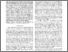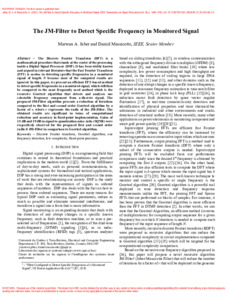RECHERCHER

# The JM-Filter to detect specific frequency in monitored signal

### Téléchargements

Téléchargements par mois depuis la dernière année

Plus de statistiques...

Jaber, M. A. et Massicotte, D. (2021). The JM-Filter to detect specific frequency in monitored signal. IEEE Transactions on Signal Processing, 69 . p. 1468-1476. ISSN 1941-0476 DOI 10.1109/TSP.2021.3053509Prévisualisation PDF Télécharger (570kB) | Prévisualisation

## Résumé

The Discrete Fourier Transform (DFT) is a mathematical procedure that stands at the center of the processing inside a digital signal processor. It has been widely known and argued in relevant literature that the Fast Fourier Transform (FFT) is useless in detecting specific frequencies in a monitored signal of length N because most of the computed results are ignored. In this paper, we present an efficient FFT-based method to detect specific frequencies in a monitored signal, which will then be compared to the most frequently used method which is the recursive Goertzel algorithm that detects and analyses one selectable frequency component from a discrete signal. The proposed JM-Filter algorithm presents a reduction of iterations compared to the first and second order Goertzel algorithm by a factor of r, where r represents the radix of the JM-Filter. The obtained results are significant in terms of computational reduction and accuracy in fixed-point implementation. Gains of 15 dB and 19 dB in signal to quantization noise ratio (SQNR) were respectively observed for the proposed first and second order radix-8 JM-Filter in comparison to Goertzel algorithm.

Type de document: Article Discrete Fourier transform Goertzel algorithm One frequency detection Low complexity Fixed-point precision 09 mai 2022 15:49 09 mai 2022 15:49 Post-print (version corrigée et acceptée) https://depot-e.uqtr.ca/id/eprint/10129Éditer la notice